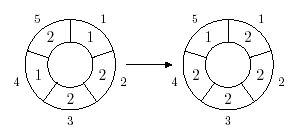Имя: Пароль: Зарегистрироваться Восстановить пароль
28/01/2023 16:00:19

#Рабочее место участника

##Задачи

123. Cellular Automaton

Ограничения: время – 3s/6s, память – 64MiB Ввод: input.txt или стандартный ввод Вывод: output.txt или стандартный выводПослать решение Blockly Посылки Темы Где Обсудить (0)

A cellular automaton is a collection of cells on a grid of specified shape that evolves through a number of discrete time steps according to a set of rules that describe the new state of a cell based on the states of neighboring cells. The order of the cellular automaton is the number of cells it contains. Cells of the automaton of order n are numbered from 1 to n. The order of the cell is the number of different values it may contain. Usually, values of a cell of order m are considered to be integer numbers from 0 to m – 1. One of the most fundamental properties of a cellular automaton is the type of grid on which it is computed.
In this problem we examine the special kind of cellular automaton — circular cellular automaton of order n with cells of order m. We will denote such kind of cellular automaton as n,m-automaton. A distance between cells i and j in n,m-automaton is defined as min(|i-j|;\ n-|i-j|). A d-environment of a cell is the set of cells at a distance not greater than d. On each d-step values of all cells are simultaneously replaced by new values. The new value of cell i after d-step is computed as a sum of values of cells belonging to the d-enviroment of the cell i modulo m. The following picture shows 1-step of the 5,3-automaton.The problem is to calculate the state of the n,m-automaton after k\ d-steps.
Input
The first line of the input file contains four integer numbers n,\ m,\ d, and k\ (1\ ≤\ n\ ≤\ 500,\ 1\ ≤\ m\ ≤\ 1\ 000\ 000,\ 0\ ≤\ d\ <\ n/2\ ,\ 1\ ≤\ k\ ≤\ 10\ 000\ 000). The second line contains n integer numbers from 0 to m – 1 — initial values of the automaton’s cells.
Output
Output the values of the n,m-automaton’s cells after k\ d-steps.

Sample Input #1

5 3 1 1
1 2 2 1 2


Sample Output #1

2 2 2 2 1


Sample Input #2

5 3 1 10
1 2 2 1 2


Sample Output #2

2 0 0 2 2

Source: NEERC ICPC, 2006